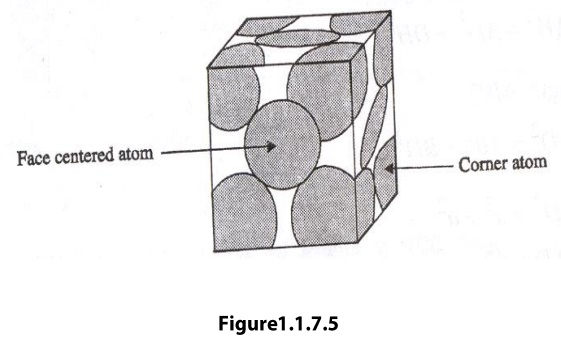Home | | Engineering Physics I | Face centered cubic (FCC) Structure

# Face centered cubic (FCC) Structure

A face centered cubic cell consists of eight corner atoms and six face centered atoms. The arrangement of atoms in face centered cubic unit is as shown in figure.

Face Centered Cubic

A face centered cubic cell consists of eight corner atoms and six face centered atoms. The arrangement of atoms in face centered cubic unit is as shown in figure.(i)                Number of atoms per unit cell

An FCC unit cell consists of eight corner atoms and each and every corner atoms is shared by eight adjacent unit cell. Therefore, each and every corner atom contributes 1/8 of its part to one unit cell. The total number of atoms by corner atom = 1/8 x 8 = 1 atom.

In addition, there are 6 atoms at the face centers of the cube. Each face-centered atom is shared by two surround unit cells. Hence, the number of face centered atoms in unit cell,

=1/2 x 6 =3 atoms.

Therefore, total number of atoms in one unit cell = 1 + 3 = 4 atoms.

(ii)             Coordination Number

In this case, there are eight atoms at the eight corners of the unit cell and 6 atom at the center of the six faces.

For any corner atom of the unit cell, the nearest atom is face-centered atom. For any corner atom, there are four face-centered atoms in its plane and four above its plane and four below its plane.

For a body centered cubic unit cell, the atomic radius can be calculated from figure as follows.(iv)           Packing Factor

The number of atoms present in an FCC unit cell is four. Therefore, the packing factor of the FCC unit cell be written as

Number of atoms per unit cell = 4This shows that 74% of the volume of an FCC unit cell is occupied by atoms and the remaining 26% volume of the unit cell is Vacant.

Thus the packing density is 74%.

Since the packing density is very high, the FCC structure has closely (or) tightly packed structure.

Study Material, Lecturing Notes, Assignment, Reference, Wiki description explanation, brief detail
Physics : Crystal Physics : Face centered cubic (FCC) Structure |